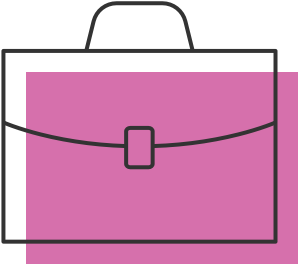Loading...

# SAS Lesson 3: Logistic Regression for a Binary Response Variable, pt 1

Course video 26 of 29

In this session, we will discuss some things that you should keep in mind as you continue to use data analysis in the future. We will also teach also you how to test a categorical explanatory variable with more than two categories in a multiple regression analysis. Finally, we introduce you to logistic regression analysis for a binary response variable with multiple explanatory variables. Logistic regression is simply another form of the linear regression model, so the basic idea is the same as a multiple regression analysis. But, unlike the multiple regression model, the logistic regression model is designed to test binary response variables. You will gain experience testing and interpreting a logistic regression model, including using odds ratios and confidence intervals to determine the magnitude of the association between your explanatory variables and response variable. You can use the same explanatory variables that you used to test your multiple regression model with a quantitative outcome, but your response variable needs to be binary (categorical with 2 categories). If you have a quantitative response variable, you will have to bin it into 2 categories. Alternatively, you can choose a different binary response variable from your data set that you can use to test a logistic regression model. If you have a categorical response variable with more than two categories, you will need to collapse it into two categories.

### About Coursera

Courses, Specializations, and Online Degrees taught by top instructors from the world's best universities and educational institutions.Join a community of 40 million learners from around the worldEarn a skill-based course certificate to apply your knowledgeGain confidence in your skills and further your career